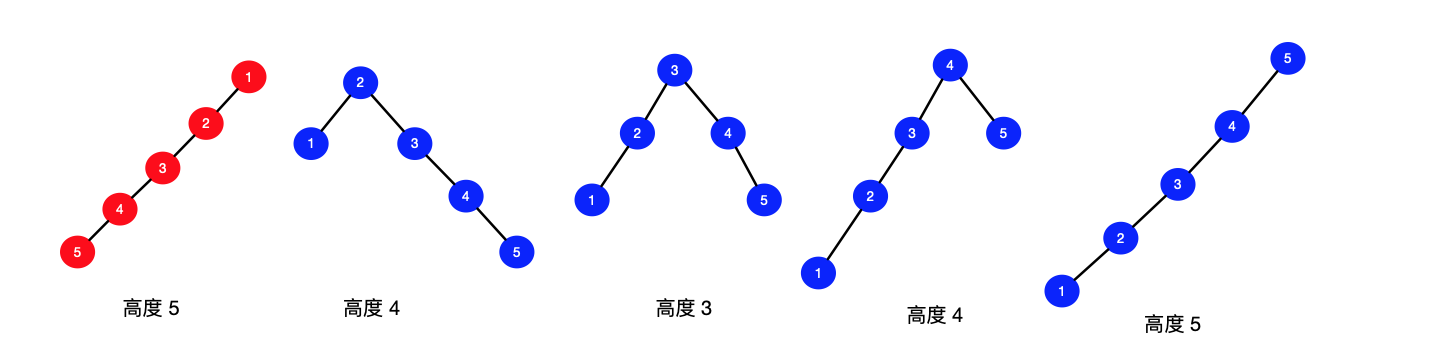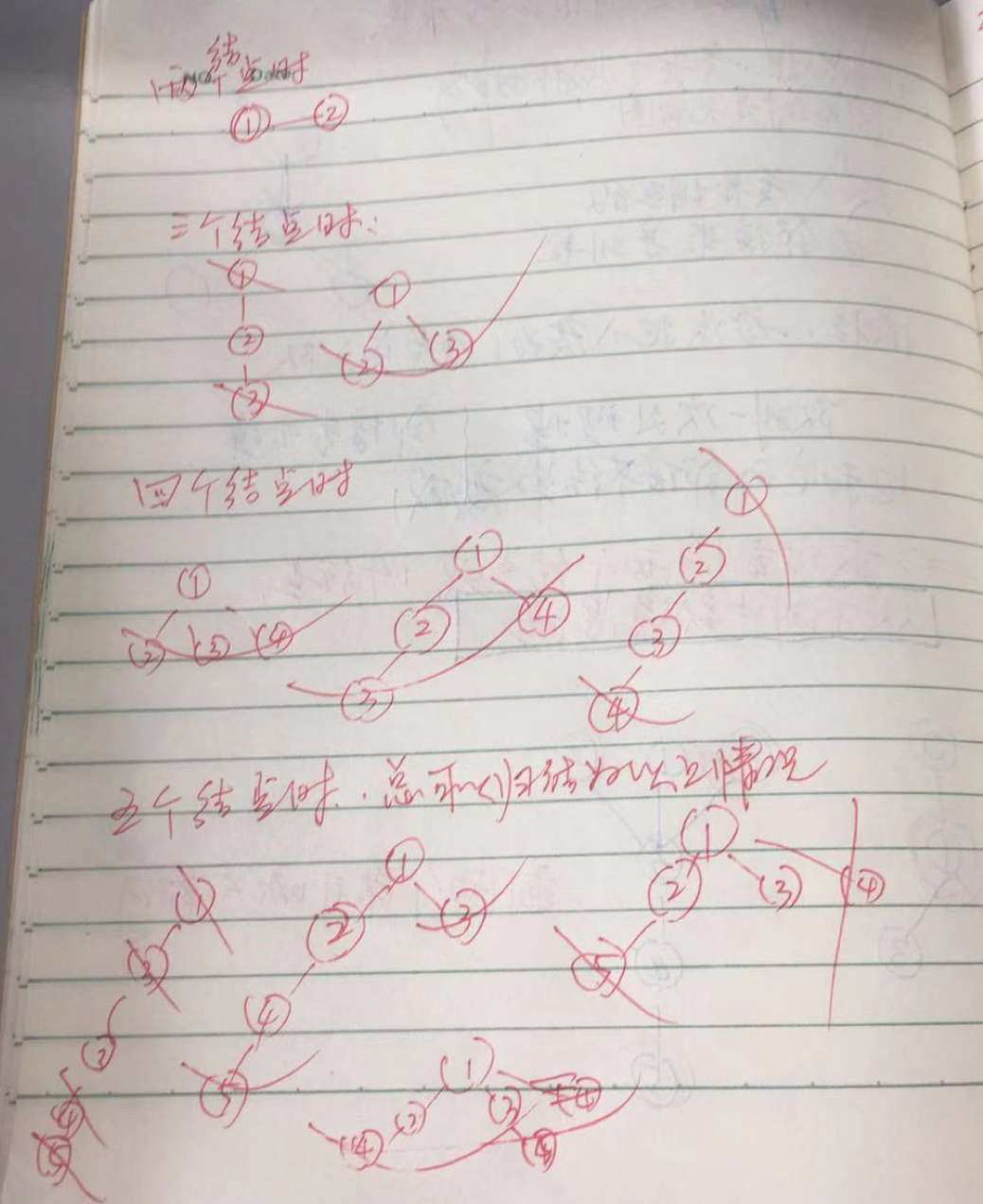# 「力扣」第 310 题：最小高度树

## 「力扣」第 310 题：最小高度树

   0
|
1
/ \
2   3 

0  1  2
\ | /
3
|
4
|
5 

### 方法：贪心算法（有的时候分析问题，需要自己动手，比看别人的思路的理解要深刻。）Java 代码：

import java.util.ArrayList;
import java.util.Arrays;
import java.util.HashSet;
import java.util.List;
import java.util.Set;

public class Solution {

public List findMinHeightTrees(int n, int[][] edges) {
List res = new ArrayList<>();
// 特判
if (n <= 2) {
for (int i = 0; i < n; i++) {
}
return res;
}

// 入度数组，每一次要把入度为 1 的结点剔除
int[] inDegrees = new int[n];

// 默认为 False，如果剔除，设置为 True
boolean[] result = new boolean[n];

// 因为是无向图，所以邻接表拿出一条边，两个结点都要存一下
// 注意：右边就不要写具体的实现类了，等到实例化的时候再写具体的实现类
// 初始化
for (int i = 0; i < n; i++) {
}

for (int[] edge : edges) {
int start = edge;
int end = edge;
inDegrees[start] += 1;
inDegrees[end] += 1;
}

// 入度为 1 的结点入队
for (int i = 0; i < n; i++) {
if (inDegrees[i] == 1) {
}
}

// 注意边界条件 n == 2 和 n == 1 是如何分析出来的
while (n > 2) {
int size = queue.size();
System.out.println(queue);
// 一次减去这么多
n -= size;
for (int i = 0; i < size; i++) {
int top = queue.removeFirst();
result[top] = true;
inDegrees[top] -= 1;
// 把它和它的邻接结点的入度全部减 1
for (Integer successor : successors) {
inDegrees[successor] -= 1;
if (inDegrees[successor] == 1) {
}
}
}
}
n = result.length;
for (int i = 0; i < n; i++) {
if (!result[i]) {
}
}
return res;
}

public static void main(String[] args) {
int[][] edges = new int[][]{{1, 0}, {1, 2}, {1, 3}};
int n = 4;
Solution solution = new Solution();
List res = solution.findMinHeightTrees(n, edges);
System.out.println(res);
}
}

Python 代码：

class Solution:

# 贪心法

def findMinHeightTrees(self, n, edges):

if n <= 2:
return [i for i in range(n)]

from collections import defaultdict
from collections import deque

in_degrees =  * n
# True 表示保留，如果设置为 False 则表示删除
res = [True] * n
# 邻接表
for edge in edges:
in_degrees[edge] += 1
in_degrees[edge] += 1

deque = deque()

for i in range(n):
if in_degrees[i] == 1:
deque.append(i)

# 根据示例，可知，最后可能剩下 1 个结点或者 2 个结点
# 或者自己画一个图也能分析出来
while n > 2:
size = len(deque)
# 一次减去当前队列这么多个结点
n -= size
for i in range(size):
top = deque.popleft()
res[top] = False

# 它和它的邻接点的入度全部减 1
successors.append(top)

for item in successors:
# 一个结点的入度重复减是没有关系的
# 我们只关心在最边界的那个结点，把它移除，所以可以认为是贪心法
in_degrees[item] -= 1

if in_degrees[item] == 1:
deque.append(item)

return [i for i in range(len(res)) if res[i]]

（本节完）

上一篇「力扣」第 343 题：整数拆分
「力扣」第 343 题：“整数拆分”题解题解地址：“贪心选择”性质的简单证明、记忆化搜索、动态规划 （Python 代码、Java 代码）。 说明：文本首发在力扣的题解版块，更新也会在第 1 时间在上面的网站中更新，这篇文章只是上面的文章的
2018-07-04「力扣」第 45 题：跳跃游戏 II
「力扣」第 45 题：跳跃游戏 II 提示：这题初学的时候很陌生，一定要多练习，经常拿出来复习一下。 链接：https://leetcode-cn.com/problems/jump-game-ii 给定一个非负整数数组，你最初位于
2018-07-02
目录